Home > A2C > Chapter 8 > Lesson 8.2.1 > Problem8-117

8-117.
1. The graph below was made by shifting the first cycle of y = sin x to the left. 8-117 HW eTool (Desmos). Homework Help ✎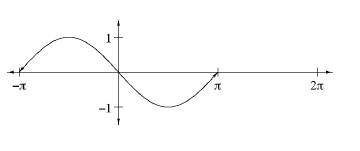1. How many units to the left was it shifted?

2. Figure out how to change the equation of y = sin x so that the graph of the new equation will look like the one in part (a). If you do not have a graphing calculator at home, sketch the graph and check your answer when you get to class.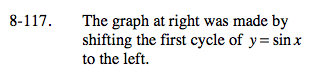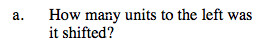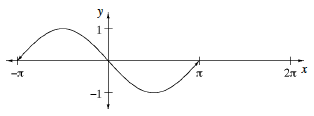It is shifted π units to the left.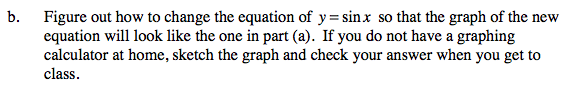In the general equation y = sin(xh) + k, which parameter moves the graph horizontally?

Use the eTool below to compare the graph with its parent graph.
Click the link at right for the full version of the eTool: A2C 8-117 HW eTool